## SGPLOT one subject at a time works; SGPANEL panelby subject doesn't work.

Greetings,

The SGPLOT code below produces this plot of transfusions (red, left Y axis) and dose (green, right Y axis) on study day (X axis):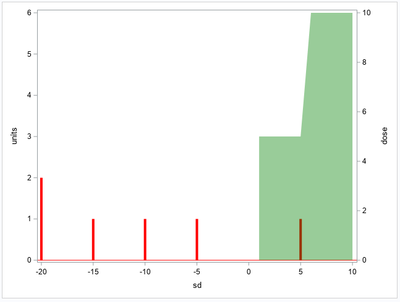The SGPANEL code produces this: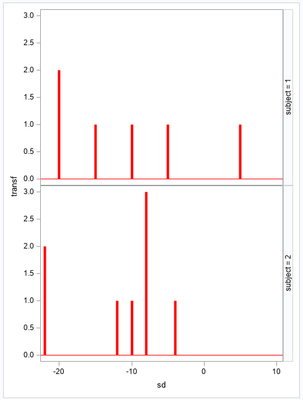My goal is to add the green dose information onto the SGPANEL procedure.  It is commented out due to errors: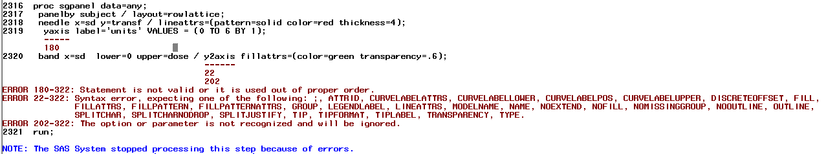I know how to cycle through all subjects using the SGPLOT, but would appreciate alternative suggestions:

1.  Should I stay with SGPANEL and fix the NEEDLE and BAND statements?  Or find other statements?

2.  Would another direction altogether be better?

John

```data any;
input subject sd dose transf;
cards;
1 -20   . 2
1 -15   . 1
1 -10   . 1
1  -5   . 1
1   1   5 .
1   2   5 .
1   3   5 .
1   4   5 .
1   5   5 1
1   6  10 .
1   7  10 .
1   8  10 .
1   9  10 .
1  10  10 .
2 -22   . 2
2 -12   . 1
2 -10   . 1
2  -8   . 3
2  -4   . 1
2   1   5 .
2   2   5 .
2   3   5 .
2   4   5 .
2   5   5 .
2   6  10 .
2   7  10 .
2   8  10 .
2   9  10 .
2  10  10 .
;
run;
proc sgplot data=any(where=(subject=1)) nolegend;
needle x=sd y=transf / lineattrs=(pattern=solid color=red thickness=4);
yaxis label='units' VALUES = (0 TO 6 BY 1);
band x=sd  lower=0 upper=dose / y2axis fillattrs=(color=green transparency=.6);
run;

proc sgpanel data=any;
panelby subject / layout=rowlattice;
needle x=sd y=transf / lineattrs=(pattern=solid color=red thickness=4);
*yaxis label='units' VALUES = (0 TO 6 BY 1);
*band x=sd  lower=0 upper=dose / y2axis fillattrs=(color=green transparency=.6);
run;```

1 ACCEPTED SOLUTION

Accepted Solutions

## Re: SGPLOT one subject at a time works; SGPANEL panelby subject doesn't work.

John

• I have used the ODS Graphics Designer to come up with the basic structure.
• Took a look at the link I mentioned earlier to see how to use the rowaxisopts and row2axisopts
• Changed the datalattice to datapanel to have the subject in one column
• Used the tickvaluesequence to be able to specify the thickmarks, default would have less of them
• The x axis will be the same for each graph, so the study days should align just well, the band starts exactly at the same position, so that is proof for that
10 REPLIES 10

## Re: SGPLOT one subject at a time works; SGPANEL panelby subject doesn't work.

Have a look here https://communities.sas.com/t5/Graphics-Programming/Can-we-get-second-y-axis-using-quot-Proc-SGpanel...

I guess you have to use GTL to get what you need.DanH_sas
SAS Super FREQ

## Re: SGPLOT one subject at a time works; SGPANEL panelby subject doesn't work.

@BrunoMueller is correct. We currently do not have "true" secondary axis support set up in PROC SGPANEL, but GTL does have this support in the DATAPANEL and DATALATTICE layouts. You should be able to re-create the same layout as SGPLOT per subject using LAYOUT DATAPANEL or DATALATTICE.

## Re: SGPLOT one subject at a time works; SGPANEL panelby subject doesn't work.

Does SGPANEL proc have a TMPLOUT option to get the GTL code as a starter like SGPLOT?

## Re: SGPLOT one subject at a time works; SGPANEL panelby subject doesn't work.

Proc SGPANEL does not have the TMPLOUT= option.

To get a starting point, one could use the ODS Graphics Designer. The code below was created using the ODS Graphics Designer.

``````proc template;
define statgraph sgdesign;
dynamic _SUBJECT _SD _TRANSF _SD2 _DOSE;
dynamic _panelnumber_;
begingraph / designwidth=773 designheight=629;
entrytitle halign=center 'Type in your title...';
entryfootnote halign=left 'Type in your footnote...';
layout datalattice rowvar=_SUBJECT / cellwidthmin=1 cellheightmin=1 rowgutter=3 columngutter=3 rowdatarange=unionall row2datarange=unionall columndatarange=unionall column2datarange=unionall headerlabeldisplay=value;
layout prototype / ;
bandplot x=_SD2 limitupper=_DOSE limitlower=0.0 / name='band' connectorder=axis extend=true;
needleplot x=_SD y=_TRANSF / name='needle' clusterwidth=0.85 grouporder=data;
endlayout;
endlayout;
endgraph;
end;
run;

proc sgrender data=SASUSER.ANY template=sgdesign;
dynamic _SUBJECT="SUBJECT" _SD="SD" _TRANSF="TRANSF" _SD2="SD" _DOSE="DOSE";
run;
``````

## Re: SGPLOT one subject at a time works; SGPANEL panelby subject doesn't work.

Bruno, Dan, and Reeza, thanks for your responses.  I appreciate your suggestions.

1.  I'm still not getting exactly what I want, which is (a) two independent vertical axes and (b) multiple panels per page.  It seems:

a.  GTL is probably the way to go to gain maximum flexibility.  I need to investigate more (I welcome further suggestions).

b.  While %sgdesign; is a useful tool to assist building graphs, I do not see multiple y axes as an option.

2.  The SGRENDER procedure below generates these two plots (using the same data as last week):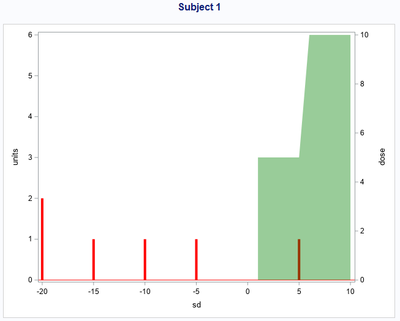a.  The PROC SGPLOT TMPLOUT= option generates the PROC TEMPLATE code.  The TMPLOUT= option is no longer supported for PROC SGPANEL.

b.  The PROC SGRENDER code is executed one subject at a time to generate these plots, but this generates one plot per page.

c.  With a better understanding of the GTL DATAPANEL or DATALATTICE statements, perhaps the PROC TEMPLATE could be modified to avoid this limitation.

``````proc sgplot data=any(where=(subject=1)) NOAUTOLEGEND tmplout="&path.a2.txt";
needle x=sd y=transf / lineattrs=(pattern=solid color=red thickness=4);
yaxis label='units' VALUES = (0 TO 6 BY 1);
band x=sd lower=0 upper=dose / y2axis fillattrs=(color=green transparency=.6);
run;* cancel;

* This is a2.txt which is output from the tmplout option above. *;
proc template;
define statgraph sgplot;
begingraph / collation=binary subpixel=on;
layout overlay / y2axisopts=(labelFitPolicy=Split) yaxisopts=( Label="units" labelFitPolicy=Split type=linear linearopts=( tickvaluelist=( 0 1 2 3 4 5 6 ) viewmin=0 viewmax=6 ) ) y2axisopts=(labelFitPolicy=Split);
NeedlePlot X='sd'n Y='transf'n / primary=true Lineattrs=( Color=CXFF0000 Pattern=1 Thickness=4) LegendLabel="transf" NAME="NEEDLE";
BandPlot X='sd'n LimitUpper='dose'n LimitLower=0 / yaxis=y2 FillAttrs=GraphConfidence(Color=CX008000 Transparency=0.6) LegendLabel="Band" NAME="BAND";
endlayout;
endgraph;
end;
run;

* Create macro variable containing all levels of variable subject. *;
proc sql noprint;
select distinct subject
into :sub separated by ' '
from work.any;
quit;
%*put _all_;

%macro loop;
* Cycle through all subjects. Use loop macro to avoid open code issue. *;
%let i=1;
%let ids=%scan(&sub,&i.,%str( ));
%do %while (%length(&ids.) GT 0);
title "Subject &ids.";
proc sgrender data=work.ANY(where=(subject=&ids.)) template=sgplot;
run;
title;
%let i=%eval(&i.+1);
%let ids=%scan(&sub,&i.,%str( ));
%end;
%mend loop;
%loop;``````

## Re: SGPLOT one subject at a time works; SGPANEL panelby subject doesn't work.

Give this program a try (uses your data). It shows how to have two y axis, you control them using the rowaxisopts= and row2axisopts=

``````
ods path
(prepend) work.mytemplates (update)
;
ods path show;

proc template;
define statgraph sgdesign;
dynamic _SUBJECT _SD _TRANSF _DOSE;
dynamic _panelnumber_;
begingraph / designwidth=1200 designheight=900;
entrytitle halign=center 'Type in your title...';
entryfootnote halign=left 'Type in your footnote...';
layout datapanel classvars=(_SUBJECT) /
columns=1

/* this is the x axis */
columnaxisopts=(
display=standard
type=linear
linearopts=(
integer=true
tickvaluesequence=(start=-25 end=10 increment=5)
)
griddisplay=on
)
/* this is the Y axis */
rowaxisopts=(
type=linear display=standard label="units"
linearopts=(integer=true viewmin=0 viewmax=6 )
)
/* this is the y2 axis */
row2axisopts=(type=linear display=standard );
;
layout prototype /;
needleplot x=_SD y=_TRANSF / name='needle' Lineattrs=( Color=red Pattern=1 Thickness=4);
bandplot x=_SD limitlower=0 limitupper=_DOSE / name='band' yaxis=y2 fillattrs=(color=green transparency=0.6);
endlayout;
endlayout;
endgraph;
end;
run;

proc sgrender data=ANY template=sgdesign;
dynamic _SUBJECT="SUBJECT" _SD="SD" _TRANSF="TRANSF" _DOSE="DOSE";
run;``````

## Re: SGPLOT one subject at a time works; SGPANEL panelby subject doesn't work.

Bruno,

This works perfectly on my test data.  Thank you.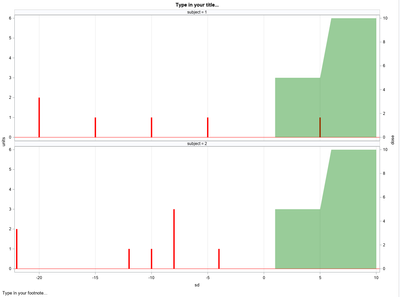I still have some work to do to get this working on my real data.  (Sorry, I should have created more robust test data, huh?  I will if that would help.)

Would you please let me know your process in coming up with this code?  This will let me know how much I need to dig into GTL options vs letting some tool write the code.  Remaining issues I can see now:

1.  X-axis issues:

a.  I can only get a non-blank plot to generate by altering the tickvaluesequence start and end values.  Presumably if I generate GTL code using my real data these start and end values will be populated automatically, though I would probably generate these dynamically so that the code can be reused on any data.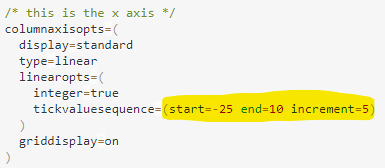b.  Even with an appropriate start and stop supplied for my real data:

i.  sd (study day) values do not appear on the x axis, and

ii.  each plot uses an independent scale meaning that study day 1 is not aligned for each plot (as the test data produced).

Thanks again.  I have a lot to learn and appreciate your help.

## Re: SGPLOT one subject at a time works; SGPANEL panelby subject doesn't work.

John

• I have used the ODS Graphics Designer to come up with the basic structure.
• Took a look at the link I mentioned earlier to see how to use the rowaxisopts and row2axisopts
• Changed the datalattice to datapanel to have the subject in one column
• Used the tickvaluesequence to be able to specify the thickmarks, default would have less of them
• The x axis will be the same for each graph, so the study days should align just well, the band starts exactly at the same position, so that is proof for that

## Re: SGPLOT one subject at a time works; SGPANEL panelby subject doesn't work.

Bruno,

Thanks for answering all my questions.  Your code DOES work with my real data after all.  I mistakenly used a date-formatted variable for the x-axis rather than the numeric Study Day variable I had prepared for this purpose.  That is why I wasn't getting what I expected.

## Re: SGPLOT one subject at a time works; SGPANEL panelby subject doesn't work.

Glad I could help and thanks for the feedback on the data
Discussion stats
• 10 replies
• 741 views
• 11 likes
• 4 in conversation